### Home > APCALC > Chapter 8 > Lesson 8.1.4 > Problem8-47

8-47.

Given: y2 = xx3 : Homework Help ✎

1. Write an equation for $\frac { d y } { d x }$.

2. For what value of y is there a vertical tangent to the graph?

3. For what values of x are there vertical tangents to the graph?

4. Write an equation for $\frac { d ^ { 2 } y } { d x ^ { 2 } }$.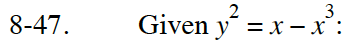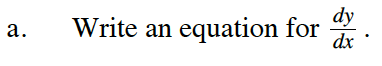Implicit differentiation.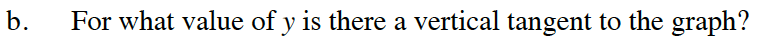Determine all y-values in which the denominator of the derivative is 0.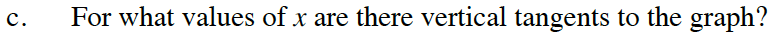Use the original function to determine the corresponding x-value for each y-value you found in part (b).

There will be three values of x that work. That means there will be three coordinate points in which the slope is vertical: ( ____, 0 ), ( ____, 0 ) and ( ____, 0 ). (Obviously, this is NOT a function!)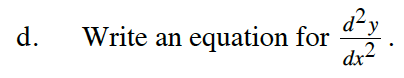The second derivative must be written in terms of x and y only.

$\text{If }\frac{dy}{dx}\text{ appears in the second derivative, substitute it with the value you found in part (a).}$